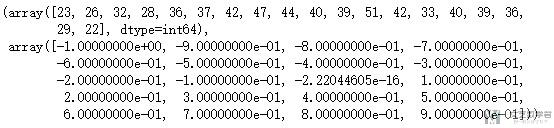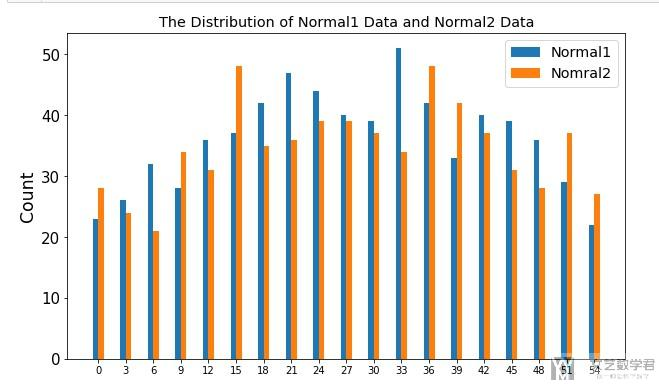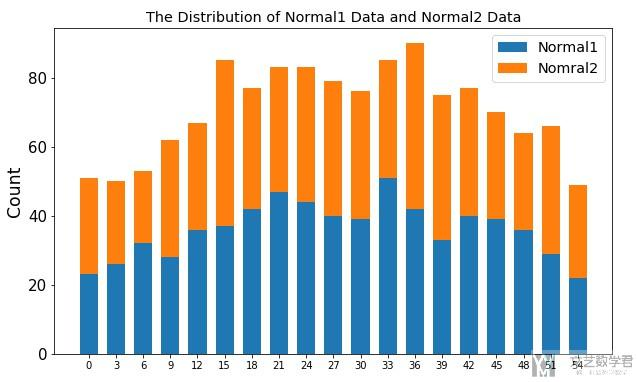# matplotlib绘图优化-使用np.histogram绘制直方图(柱状图)2019年7月12日07:47:26

3062字阅读10分12秒

## numpy.histogram的介绍

1. # 生成一些随机数
2. rng = np.random.RandomState(10)
3. data1 = rng.normal(size=1000)
4. data2 = rng.normal(size=1000)
5. # 查看np.histogram的输出
6. binRange = np.arange(-1,1,0.1)
7. print(binRange)
8. np.histogram(data1, bins=binRange)

1. [-1.00000000e+00 -9.00000000e-01 -8.00000000e-01 -7.00000000e-01
2.  -6.00000000e-01 -5.00000000e-01 -4.00000000e-01 -3.00000000e-01
3.  -2.00000000e-01 -1.00000000e-01 -2.22044605e-16  1.00000000e-01
4.   2.00000000e-01  3.00000000e-01  4.00000000e-01  5.00000000e-01
5.   6.00000000e-01  7.00000000e-01  8.00000000e-01  9.00000000e-01]

• 第一个部分表示每个区间的统计个数；
• 第二个部分表示每个区间的边界；### 显示百分比

1. rng=np.random.RandomState(10)
2. data1=rng.normal(size=1000)
3. bin_range=[-1,0,2] # 设置区间, 分别是[-1,0]和[0,2]
4. # 计算每一个区间所占的百分比
5. hist,_ = np.histogram(data1, bin_range, density=True)
6. hist
7. """
8. array([0.42822678, 0.28588661])
9. """

1. np.sum(hist*np.array([1,2]))
2. """
3. 1.0
4. """

## np.histogram结合plt.bar绘制直方图

### 绘制多个直方图

1. hist1,_ = np.histogram(data1, bins=binRange)
2. hist2,_ = np.histogram(data2, bins=binRange)
3. # 绘制图像
4. fig, ax1 = plt.subplots()
5. fig.set_size_inches(10, 6)
6. plt.set_cmap('RdBu')
7. x = np.arange(len(binRange)-1)*3
8. w=0.3
9. # 绘制多个bar在同一个图中, 这里需要控制width
10. plt.bar(x-w, hist1, width = 2*w, align='center')
11. plt.bar(x+w, hist2, width = 2*w, align='center')
12. # 设置坐标轴的标签
13. ax1.yaxis.set_tick_params(labelsize=15) # 设置y轴的字体的大小
14. ax1.set_xticks(x) # 设置xticks出现的位置
15. # 设置坐标轴名称
16. ax1.set_ylabel("Count", fontsize='xx-large')
17. # 设置标题
18. ax1.set_title('The Distribution of Normal1 Data and Normal2 Data', fontsize='x-large')
19. # 设置图例
20. plt.legend(('Normal1','Nomral2'),fontsize = 'x-large', loc='upper right')
21. plt.show()### 绘制堆叠直方图

1. hist1,_ = np.histogram(data1, bins=binRange)
2. hist2,_ = np.histogram(data2, bins=binRange)
3. # 绘制图像
4. fig, ax1 = plt.subplots()
5. fig.set_size_inches(10, 6)
6. plt.set_cmap('RdBu')
7. x = np.arange(len(binRange)-1)*3
8. w=1
9. # 绘制多个bar在同一个图中, 这里需要控制width
10. plt.bar(x, hist1, width = 2*w, align='center')
11. plt.bar(x, hist2, width = 2*w, align='center', bottom=hist1)
12. # 设置坐标轴的标签
13. ax1.yaxis.set_tick_params(labelsize=15) # 设置y轴的字体的大小
14. ax1.set_xticks(x) # 设置xticks出现的位置
15. # 设置坐标轴名称
16. ax1.set_ylabel("Count", fontsize='xx-large')
17. # 设置标题
18. ax1.set_title('The Distribution of Normal1 Data and Normal2 Data', fontsize='x-large')
19. # 设置图例
20. plt.legend(('Normal1','Nomral2'),fontsize = 'x-large', loc='upper right')
21. plt.show()• 微信公众号
• 关注微信公众号
•• QQ群
• 我们的QQ群号
•• 本文由 发表于 2019年7月12日07:47:26
• 转载请务必保留本文链接：https://mathpretty.com/10768.html